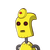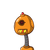# 3cm3. In the figure the perimeter of triangle ABC is6cmA) 11 cmB) 15 cmC) 22 cmD)

3cm
3. In the figure the perimeter of triangle ABC is
6cm
A) 11 cm
B) 15 cm
C) 22 cm
D) 30cm
P
2cm B​

### 2 thoughts on “<br /><br />3cm<br />3. In the figure the perimeter of triangle ABC is<br />6cm<br />A) 11 cm<br />B) 15 cm<br />C) 22 cm<br />D)”

1.30 is the answer for this questions

2.By the theorem of length of tangents from an external point to a circle,

measure of PA = PC

Therefore, PC = PA = 4 cm

Now PR = 9 cm

and PR = PC + CR

Therefore, 9 = 4 + CR

CR = 9 – 4 = 5 cm

From the theorem of tangents again,

CR = BR = 5 cm

and BQ = AQ = 6 cm

Now we know the length of PR = 9 cm

length o PQ = PA + AQ = 4 + 6 = 10 cm

and length of QR = QB + BR = 6 + 5 = 11 cm

Perimeter of ΔPQR = PQ + QR + PR

= 10 + 11 + 9

= 30 cm

Therefore, perimeter of ΔPQR is 30 cm.